# Common Core: High School - Geometry : Construct Geometric Figures: CCSS.Math.Content.HSG-CO.D.12

## Example Questions

← Previous 1

### Example Question #131 : Congruence

How is a square altered to result in a rhombus?

Change the length of the sides

A square can never be a rhombus

Rotate the squareChange the interior angles.

Change the vertical distance between the two horizontal lines.

Change the interior angles.

Explanation:

Both squares and rhombi are quadrilaterals and parallelograms. Quadrilaterals are four sided figures and parallelograms are figures that have opposite sides that are parallel.

Squares by definition contain fourangles; rhombi on the other hand have two sets of opposite congruent angles. Therefore, for a square to to altered into a rhombus, the interior angles must be altered.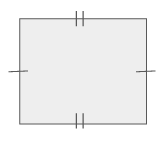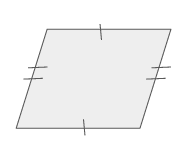### Example Question #1 : Construct Advanced Geometric Figures

Given the following ray, construct an angle that is.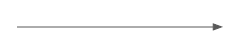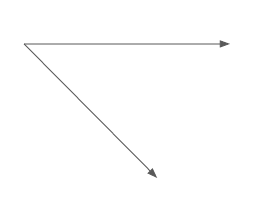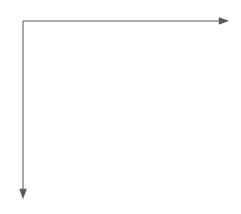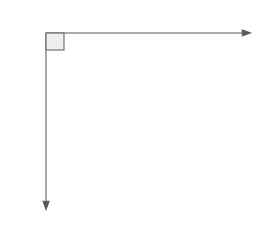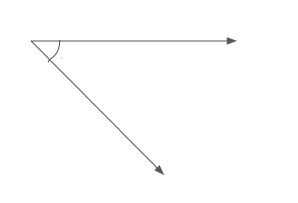Explanation:

In order to construct aangle using a ray, first recall what aangle and ray are.

An angle in the most basic terms is when two rays having the same vertex, are going in different directions.

Now, recall that aangle occurs when the lines or rays of the angle intersect perpendicularly.

Since the problem gives the starting ray,which is a horizontal ray, then to create aangle, a vertical ray needs to be constructed from the same vertex. To identify aangle it is common practice to draw a square at the vertex to show the angle measurement. This results in the final solution.### Example Question #1 : Construct Advanced Geometric Figures

Given a line, what is the first step taken to construct a parallel line?

Find the-intercept of the given line.

Identify the two lines intersection point.

Find the-intercept of the given line.

Calculate the slope of the given line.

Take the negative reciprocal of the given line's slope.

Calculate the slope of the given line.

Explanation:

To identify the first step that must be taken when constructing a parallel line, there is some information about the given line that needs to be clearly established first.

First recall what it means to be parallel. For lines to be parallel, their slopes must be equal and they must have differentandintercepts. If two lines have the same intercepts and the same slope they are not parallel lines, they are the same line. Parallel lines mean that two lines will never intersect.

From here, look at the possible answers to decide which one is best.

"Identify the two lines intersection point." by definition of parallel lines this is false since parallel lines never intersect.

"Find the-intercept of the given line." and "Find the-intercept of the given line." could possible be considered among the steps to constructing parallel lines but not necessarily.

"Take the negative reciprocal of the given line's slope." Taking this would give the slope of a perpendicular line.

"Calculate the slope of the given line." in order to construct a parallel line, the slope must first be calculated on the given line. Therefore, this is the best answer.

### Example Question #2 : Construct Advanced Geometric Figures

Given a line, what is the first step taken to construct a perpendicular line?

Take the negative reciprocal of the given line's slope.

Find the-intercept of the given line.

Either calculate the slope of the given line or identify the intersection point of the two lines.

Calculate the slope of the given line.

Identify the two lines intersection point.

Either calculate the slope of the given line or identify the intersection point of the two lines.

Explanation:

To identify the first step that must be taken when constructing a perpendicular line, there is some information about the given line that needs to be clearly established first.

First recall what it means to be perpendicular. For lines to be perpendicular, their slopes must be opposite reciprocals of each other and they must have one point of intersection.

From here, look at the possible answers to decide which one is best.

"Find the-intercept of the given line."  doesn't necessarily help when constructing a perpendicular line.

"Take the negative reciprocal of the given line's slope." Taking this would give the slope of a perpendicular line but in order to do so the slope must be known of the given line.

"Calculate the slope of the given line." in order to construct a perpendicular line, the slope must be calculated on the given line.

"Either calculate the slope of the given line or identify the intersection point of the two lines." In order to construct a perpendicular line, the intersection point must be found and the slope of the given line must be found before the slope of the perpendicular line is found. Therefore, this is the best answer.

### Example Question #2 : Construct Advanced Geometric Figures

To construct a regular pentagon, what tools are required?

Straight Edge, Compass, Pencil, Paper

Straight Edge, Compass, Pencil, Paper, Measuring Device

Straight Edge, Compass, Pencil, Measuring Device

Compass, Pencil, Paper, Measuring Device

Straight Edge, Pencil, Paper, Measuring Device

Straight Edge, Compass, Pencil, Paper, Measuring Device

Explanation:

In order to know which tools are required to construct a regular pentagon first recall the attributes of a pentagon and the possible geometric tools used to construct figures.

A regular pentagon by definition is a five sided figure. All sides of the figure are equal and every interior angle measures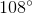.

Geometric tools used to construct figures by hand include the follow:

Paper, Pencil, Compass, Ruler, Straight Edge, Protractor, Etc.

Geometric tools used to construct figures using technology include the following:

Graphing Calculators, Computer Programs, Etc.

Since the figure in question is a regular pentagon, to construct it by hand will require the use of a compass to ensure the angles are all equaled to. Also, a straight edge is required to ensure the lines are straight and a measuring device such as a rule to ensure the side lengths are equal.

Of the possible answer choices, three list the Straight Edge, Compass, and Measuring Device. If these tools are being used then the figure is being drawn by hand therefore, pencil and paper are also required. Therefore, the correct solution is:

"Straight Edge, Compass, Pencil, Paper, Measuring Device"

### Example Question #3 : Construct Advanced Geometric Figures

Given two rays, how can a line be constructed?

Connect the vertices of the rays and have their directions pointfrom each other.

Two rays cannot be constructed into one line.

Connect the vertices of the rays and have their directions pointfrom each other.

Have their directions pointfrom each other.

Connect the vertices of the rays and have their directions pointfrom each other.

Explanation:

Recall that a ray is a line segment that has a vertex at one end and extends at the other. The extension is depicted by an arrow. In order for two rays to become one line segment the vertices must be connected and the arrows going in the opposite direction. For the rays to be going in the opposite direction they must haveangle between them.

"Connect the vertices of the rays and have their directions pointfrom each other."

### Example Question #1 : Construct Basic Geometric Figures

What must occur to construct two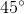angles from aangle?

Move the terminal ray of the angle up.

Move the nonterminal ray of the angle down.

Bisect thein half.

Move the terminal ray of the angle down.

Move the nonterminal ray of the angle up.

Bisect thein half.

Explanation:

To construct twoangles from aangle a bisection must occur. Recall that bisection means to cut an angle into two equal parts.

Since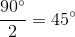that makes a bisection of theangle equal twoangles and thus is the solution.

Moving the terminal ray will not create two angles and thus it cannot be the solution.

Nonterminal is not the correct terminology and therefore, it cannot be the correct solution.

### Example Question #211 : Congruence

How are congruent lines identified in a parallelogram?

One single hash mark on one line and a double hash mark on the parallel line

Double hash marks

Either single or double hash marks

Single hash marks

Either single or double hash marks

Explanation:

Parallel lines by definition are lines that will never intersect one another. This means the slope of the lines are the same. When these lines make up a parallelogram figures that are created include squares, rectangles, diamonds, and rhombi.

Some images that depict parallelograms are as follows. Notice that either single or double hash marks can be used to identify that opposite lines are congruent.### Example Question #211 : Congruence

Given the point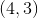, how would perpendicular lines be constructed?

Perpendicular lines are done by drawing a vertical line at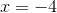and a horizontal line at.

Perpendicular lines are done by drawing a vertical line at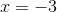and a horizontal line at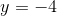.

Perpendicular lines are done by drawing a vertical line atand a horizontal line at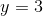.

Perpendicular lines are done by drawing a vertical line atand a horizontal line at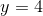.

Perpendicular lines are done by drawing a vertical line atand a horizontal line at.

Perpendicular lines are done by drawing a vertical line atand a horizontal line at.

Explanation:

To construct perpendicular lines first recall what it means to be perpendicular.

Recall that perpendicular lines intersect at one point and have opposite, reciprocal slopes.

Plotting the given point is as follows: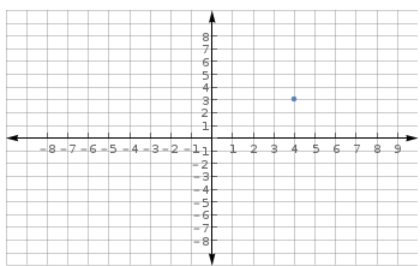Given this point, there are numerous combinations of perpendicular lines that can be made. Looking at the possible options the only pair that intersect at the pointis when a vertical line is drawn atand a horizontal line at.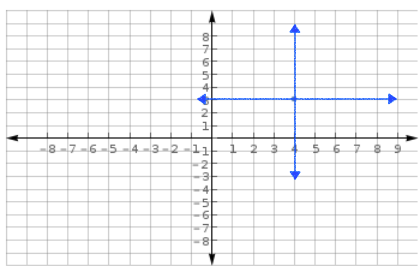### Example Question #581 : High School: Geometry

Determine whether the statement is true or false:

Lines that have fractional slopes cannot be perpendicular to other lines.

True

False

False

Explanation:

For two lines to be perpendicular they must intersect at one point and have the opposite reciprocals on each other. Having slopes that are opposite reciprocals creates aangle between the two lines which is also by definition makes the two lines perpendicular.

Therefore, a line that has a fractional slope can also have a perpendicular line. If the line in question has a slope of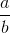then the perpendicular line would have a slope of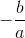.

Thus, the statement, "Lines that have fractional slopes cannot be perpendicular to other lines." is false.

← Previous 1

### All Common Core: High School - Geometry Resources